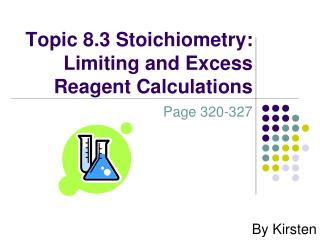DownloadDownload PresentationTopic 8.3 Stoichiometry: Limiting and Excess Reagent Calculations

# Topic 8.3 Stoichiometry: Limiting and Excess Reagent Calculations

Télécharger la présentation## Topic 8.3 Stoichiometry: Limiting and Excess Reagent Calculations

- - - - - - - - - - - - - - - - - - - - - - - - - - - E N D - - - - - - - - - - - - - - - - - - - - - - - - - - -
##### Presentation Transcript

1. Topic 8.3 Stoichiometry: Limiting and Excess Reagent Calculations Page 320-327 By Kirsten

2. What is Stoichiometry?Lets take a closer look. • A method of predicting or analyzing the quantities of the reactants and products participating in a chemical process • Three forms including gas stoichiometry, solution stoichiometry, and gravimetric stoichiometry.

3. Example of Stoichiometry • In a precipitation reaction, KOH(aq) reacts with excess Sn(NO3)2(aq) to produce a precipitate. If the mass of precipitate is 2.57 g, what mass of KOH(s) was present in the original solution?

4. First Step: Balance Equation: 2KOH(aq) + Sn(NO3) 2(aq) Sn(OH)2(s) + 2KNO3(aq)

5. Second Step: n1= 2.57 g x (1 mol/ 152.71 g) = 0.0168 mol n2= 0.0168 mol x (2/1) = 0.0337 mol m= 0.0337 mol x (56.11 mol/g) = 1.89g

6. Understanding Chemical Principles • Conservation of Mass in a Chemical Reaction • In chemical reactions the mass is conserved, meaning that the mass of the products equals the mass of the reactants. • Mass Products = Mass Reactants • There will always be an equal number of moles of each element before and after a reaction takes place

7. Identifying Reagents • Limiting Reagents • Completely consumed in a chemical reaction • Excess Reagents • More is present than is necessary to react with the limiting reagent • The limited reagent is the reagent that is being analyzed in a quantitative analysis where limiting and excess reagents are present.

8. How to find Limiting Regent: • Ensure that all masses given are in moles. • Take the molar amounts from each substance and divide by the coefficient of that substance in the balanced equation. • The smaller number will be the limiting reagent.

9. Example 1: • 300 mL of 0.100 mol/L of BaCl2(aq) and 200 mL of 0.110 mol/L of Na2CO3(aq) are mixed. What is the limiting reagent in the reaction? Answer: Na2CO3

10. Why? BaCl2(aq) + Na2CO3(aq) BaCO3(s) + 2NaCl(aq) With a 1:1 mole ratio of reactants he species present in least amount is the limiting reagent. nBaCl2= 300mL x (0.100mol/L) =30.0 mmol nNa2CO3= 200 mL x (0.110mol/L) = 22.0 mmol Therefore Na2CO3(aq) is the limiting reagent

11. Example 2: • 100.0 g of iron (III) chloride and 50.00g of hydrogen sulfide react. What is the limiting reagent? 2FeCl3 + 3H2S Fe2S3 + 6HCl

12. Answer: • Iron(III) chloride 100g/ 162.204 g/mol =0.6165 mol • Hydrogen Sulfide 50.00g/ 34.081 g/mol =1.467 mol 1.467 mol/ 3 =0.489 • Iron (III) chloride is the limiting reagent

13. Example 3: • In an experiment, 26.8 g of iron(III) chloride in solution is combined with 21.5 g of sodium hydroxide in solution. Which reactant is in excess, and by how much? What mass of each product will be obtained?

14. Answer: FeCl3(aq) + 3NaOH(aq) Fe(OH)3(s) + 3NaCl(aq) 26.8 g 21.5g m m 162.20g/mol 40.00 g/mol 106.88 g/mol 58.44 g/mol nFeCl3 = 26.8 g x (1mol/ 162.20g0 =0.165 mol nNaOH = 21.5 g x (1 mol / 40.00g) = 0.538 mol

15. Answer: nNaOH=0.165 mol x (3/1) =0.496 nNaOH= 0.538 mol - 0.496 mol = 0.042 mol mNaOH = 0.042 mol x (40.00g/1 mol) = 1.7g

16. Answer: mFe(OH)3= 0.165 molFeCl3 x (1 mol Fe(OH)3/1 molFeCl3) x (106.88 gFe(OH)3/ 1 mol Fe(OH)3) = 17.7 g Fe(OH)3 m NaCl = 0.165 molFeCl3 x (3 mol NaCl/ 1 mol FeCl3) x (58.44 g NaCl / 1 mol NaCl) = 29.0 NaCl Therefore sodium hydroxide is in excess by 1.7 g, the mass of iron (III) hydroxide produced is 17.7 g and the mass of sodium chloride produced is 29.0g.

17. Theoretical yields Vs. Actual yields • The theoretical yield of a chemical reaction is the amount of product formed if all of the limiting reagent reacts. • Calculated using stoichiometry. • The actual yield is the actual quantity of products formed after a chemical reaction. • Usually the theoretical yield will be greater than the actual yield that is produced.

18. Reasons for Discrepancy • The actual yield of a chemical reaction is usually less than the theoretical yield for these reasons • Purity of chemicals being used • Errors in measurements • Experimental factors that may have lead to loss of reactants

19. % Error Calculations: • A reasonable quantity of reasonable excess reagent is 10%

20. Example 4: • You decide to test the method of stoichiometry using the reaction of 2.00 g of copper(II) sulfate in solution with an excess of sodium hydroxide in solution. What would be a reasonable mass of sodium hydroxide to use? CuSO4(aq) + 2NaOH(aq) Cu(OH)2(aq) + Na2SO4(aq) 2.00 g m 159.62 g/mol 40.00 g/mol

21. Answer: nCuSO4 = 2.00g x (1 mol/159.62 g) =0.0125 mol nNaOH =0.0125 mol x (2/1) =0.0251 mol mNaOH = 0.0251 mol x (40g/1mol) = 1.00 g Now add 10% to this amount to determine the reasonable mass of sodium hydroxide to use. 1.00 g + 0.10g =1.10g

22. For More Information: • Nelson Chemistry Text • Pages 320-327 • The Key- Chemistry 20 • D2L Web Lessons